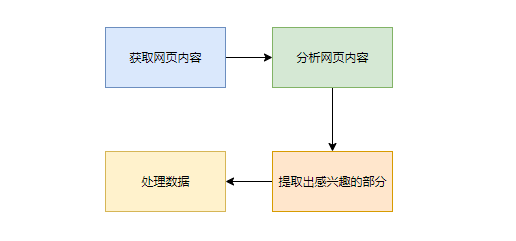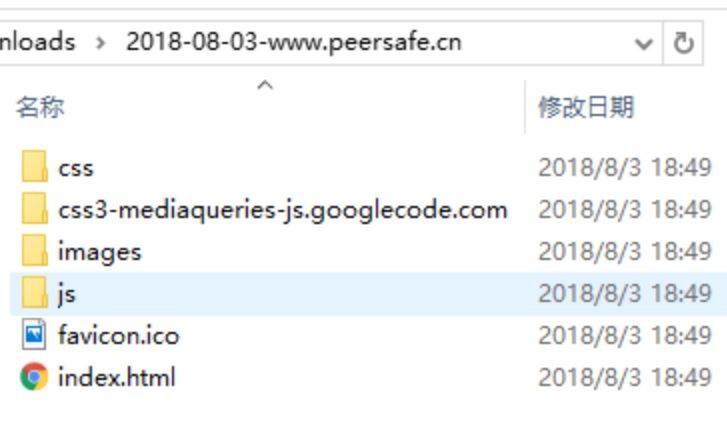# 如何利用Python抓取静态网站及内部资源

XAMPP相关 38浏览

## 遇到的需求

1. 打开`chrome`的控制台，进入`Application`选项
2. 找到`Frames`选项，找到html文件，再`右键Save As...`
3. 手动创建本地的`js/css/images`目录
4. 依次打开`Frames`选项下的`Images/Scripts/Stylesheets`，一个文件就要`右键Save As...`

1. 手工操作，麻烦费时
2. 一不小心就忘记保存哪个文件
3. 难以处理路径之间的关系，比如一张图片`a.jpg`, 它在`html`中的引用方式是`images/banner/a.jpg`，这样我们以后还要手动去解决路径依赖关系

## 前置知识储备

### 爬虫的概念1. 自己抓取网页内容，然后自己实现分析过程
2. 用别人写好的爬虫框架，比如`Scrapy`

### 正则表达式

#### 概念

``````function trim(value) {
return value.replace(/^\s+|\s+\$/g, '')
}

// 输出 => "Python爬虫"
trim(' Python爬虫 ');``````

#### 正则表达式中的元字符

##### 匹配单个字符的元字符

• `.`代表匹配一个任意字符，除了`\n`(换行符)，比如可以匹配任意的字母数字等等
• `[...]`表示字符组，里面可以有任意字符，它只会匹配当中的任意一个，比如`[abc]`可以匹配`a``b``c`，这里值得注意的是，字符组里面的元字符有时候会被当成是普通字符，比如`[-*?]`等等，它代表的仅仅是`-``*``?`，而不是`-代表区间``*代表0到无穷次匹配``?代表0或1次匹配`
• `[^...]``[...]`的含义相反，它的意思是匹配一个不属于`[...]`里面的字符，而不是不匹配`[...]`里面的字符，这两种说法虽然细微但是有很大差别，前者规定一定要匹配一个字符，这个切记。

##### 提供计数功能的元字符

• `*`代表匹配`0次到无穷次`，可以不匹配任何字符
• `+`代表匹配`1次到无穷次`，至少匹配1次
• `?`代表匹配`0次或1次`
• `{min, max}`代表匹配`min次到max次`，如`a{3, 5}`表示a至少匹配3-5次

##### 提供位置的元字符

• `^`代表匹配字符串开头，如`^a`表示a要出现在字符串开头，bcd则不匹配
• `\$`代表匹配字符串结尾, 如`A\$`表示A要出现在字符串结尾，ABAB则不匹配
##### 其他元字符

• `|`代表一个范围，可以匹配任意的子表达式，比如`abc|def`可以匹配abc或者def，不匹配abd
• `(...)`代表分组，它的作用有界定子表达式的范围和与提供功能的元字符相结合，比如`(abc|def)+`代表可以匹配1次或1次以上的abc或者defdef，如abcabcabc，def
• `\i`代表反向引用，i可以为1/2/3等整数，它的含义是指向上一个()里面匹配的内容。比如匹配`(abc)+(12)*\1\2`，如果匹配成功的话，`\1`的内容是abc，`\2`的内容是12或者空。反向引用通常用在匹配`""`或者`''`

#### 环视

• `肯定顺序环视`：先找到环视中的文本在右侧出现的初始位置，然后从匹配到的右侧文本的最左的位置开始匹配字符
• `否定顺序环视`：先找到环视中的文本在右侧没有出现的初始位置，然后从匹配到的右侧文本的最左的位置开始匹配字符
• `肯定逆序环视`：先找到环视中的文本在左侧出现的初始位置，然后从匹配到的左侧文本的最右的位置开始匹配字符
• `否定逆序环视`：先找到环视中的文本在左侧没有出现的初始位置，然后从匹配到的左侧文本的最右的位置开始匹配字符
##### 肯定顺序环视

``````import re

reg1 = r'(?=hello)he'

print(re.search(reg1, 'hello world'))
print(re.search(reg1, 'hell world hello'))
print(re.search(reg1, 'hell world'))

# 输出结果
<_sre.SRE_Match object; span=(0, 2), match='he'>
<_sre.SRE_Match object; span=(11, 13), match='he'>
None``````

##### 否定顺序环视

``````import re

reg2 = r'(?!hello)he'

print(re.search(reg2, 'hello world'))
print(re.search(reg2, 'hell world hello'))
print(re.search(reg2, 'hell world'))

# 输出结果
None
<_sre.SRE_Match object; span=(0, 2), match='he'>
<_sre.SRE_Match object; span=(0, 2), match='he'>``````
##### 肯定逆序环视

``````import re

reg3 = r'(?<=hello)-python'
print(re.search(reg3, 'hello-python'))
print(re.search(reg3, 'hell-python hello-python'))
print(re.search(reg3, 'hell-python'))

# 输出结果
<_sre.SRE_Match object; span=(5, 12), match='-python'>
<_sre.SRE_Match object; span=(17, 24), match='-python'>
None``````
##### 否定逆序环视

``````import re

reg3 = r'(?<=hello)-python'
print(re.search(reg3, 'hello-python'))
print(re.search(reg3, 'hell-python hello-python'))
print(re.search(reg3, 'hell-python'))

# 输出结果
<_sre.SRE_Match object; span=(5, 12), match='-python'>
<_sre.SRE_Match object; span=(17, 24), match='-python'>
None``````

#### 捕获分组

``````import re

reg7 = r'hello,([a-zA-Z0-9]+)'
print(re.search(reg7, 'hello,world').groups())
print(re.search(reg7, 'hello,world').group(1))
print(re.search(reg7, 'hello,python').groups())
print(re.search(reg7, 'hello,python').group(1))

# 输出结果
('world',)
world
('python',)
python``````

#### 贪婪匹配

``````import re

reg5 = r'hello.*world'
print(re.search(reg5, 'hello world,hello python,hello world,hello javascript'))

# 输出结果
<_sre.SRE_Match object; span=(0, 36), match='hello world,hello python,hello world'>``````

``````import re

reg5 = r'hello.*world'
reg6 = r'hello.*?world'
print(re.search(reg5, 'hello world,hello python,hello world,hello javascript'))
print(re.search(reg6, 'hello world,hello python,hello world,hello javascript'))

# 输出结果
<_sre.SRE_Match object; span=(0, 36), match='hello world,hello python,hello world'>
<_sre.SRE_Match object; span=(0, 11), match='hello world'>``````

## 进入开发

### 我们想实现的最终效果### 开发流程

#### 获取网页内容

``````url = 'http://www.peersafe.cn/index.html'

# 读取网页内容
webPage = urllib.request.urlopen(url)
content = data.decode('UTF-8')
print('> 网站内容抓取完毕，内容长度：', len(content))``````

``````# python-spider-downloads是我们要放置的目录
# 这里推荐使用os模块来获取当前的目录或者拼接路径

``````{'baseUrl': 'http://www.peersafe.cn', 'fullPath': 'http://www.peersafe.cn/', 'protocol': 'http://', 'domain
': 'www.peersafe.cn', 'path': '/', 'fileName': 'index.html', 'ext': 'html', 'params': ''}``````

``````REG_URL = r'^(https?://|//)?((?:[a-zA-Z0-9-_]+\.)+(?:[a-zA-Z0-9-_:]+))((?:/[-_.a-zA-Z0-9]*?)*)((?<=/)[-a-zA-Z0-9]+(?:\.([a-zA-Z0-9]+))+)?((?:\?[a-zA-Z0-9%&=]*)*)\$'

regUrl = re.compile(REG_URL)

# ...

'''

'''
def parseUrl(url):
if not url:
return

res = regUrl.search(url)
# 在这里，我们把192.168.1.109:8080的形式也解析成域名domain，实际过程中www.baidu.com等才是域名，192.168.1.109只是IP地址
# ('http://', '192.168.1.109:8080', '/abc/images/111/', 'index.html', 'html', '?a=1&b=2')
if res is not None:
path = res.group(3)
fullPath = res.group(1) + res.group(2) + res.group(3)

if not path.endswith('/'):
path = path + '/'
fullPath = fullPath + '/'
return dict(
baseUrl=res.group(1) + res.group(2),
fullPath=fullPath,
protocol=res.group(1),
domain=res.group(2),
path=path,
fileName=res.group(4),
ext=res.group(5),
params=res.group(6)
)

'''

eg:
resourcePath => /a/b/c/ or a/b/c

'''
def resolvePath(basePath, resourcePath):
# 解析资源路径
res = resourcePath.split('/')
# 去掉空目录 /a/b/c/ => [a, b, c]
dirList = list(filter(lambda x: x, res))

# 目录不为空
if dirList:
# 拼接出绝对路径
resourcePath = reduce(lambda x, y: os.path.join(x, y), dirList)
dirStr = os.path.join(basePath, resourcePath)
else:
dirStr = basePath

return dirStr``````

``````# 首先创建这个站点的文件夹
urlDict = parseUrl(url)
print('分析的域名：', urlDict)
domain = urlDict['domain']

filePath = time.strftime('%Y-%m-%d', time.localtime()) + '-' + domain
# 如果是192.168.1.1:8000等形式，变成192.168.1.1-8000，:不可以出现在文件名中
filePath = re.sub(r':', '-', filePath)
SAVE_PATH = os.path.join(SAVE_PATH, filePath)

# 读取网页内容
webPage = urllib.request.urlopen(url)
content = data.decode('UTF-8')
print('> 网站内容抓取完毕，内容长度：', len(content))

# 把网站的内容写下来
pageName = ''
if urlDict['fileName'] is None:
pageName = 'index.html'
else:
pageName = urlDict['fileName']

pageIndexDir = resolvePath(SAVE_PATH, urlDict['path'])
if not os.path.exists(pageIndexDir):
os.makedirs(pageIndexDir)

pageIndexPath = os.path.join(pageIndexDir, pageName)
print('主页的地址:', pageIndexPath)
f = open(pageIndexPath, 'wb')
f.write(data)
f.close()``````

#### 提取有用的资源链接

``````REG_RESOURCE_TYPE = r'(?:href|src|data\-original|data\-src)=["\'](.+?\.(?:js|css|jpg|jpeg|png|gif|svg|ico|ttf|woff2))[a-zA-Z0-9\?\=\.]*["\']'

# re.S代表开启多行匹配模式
regResouce = re.compile(REG_RESOURCE_TYPE, re.S)

# ...

# 解析网页内容，获取有效的链接
# content是上一步读取到的网页内容
contentList = re.split(r'\s+', content)
resourceList = []
for line in contentList:
resList = regResouce.findall(line)
if resList is not None:
resourceList = resourceList + resList``````

#### 下载资源

``````# ./static/js/index.js
# /static/js/index.js
# static/js/index.js
# //abc.cc/static/js
# http://www.baidu/com/static/index.js
if resourceUrl.startswith('./'):
resourceUrl = urlDict['fullPath'] + resourceUrl[1:]
elif resourceUrl.startswith('//'):
resourceUrl = 'https:' + resourceUrl
elif resourceUrl.startswith('/'):
resourceUrl = urlDict['baseUrl'] + resourceUrl
elif resourceUrl.startswith('http') or resourceUrl.startswith('https'):
# 不处理，这是我们想要的url格式
pass
elif not (resourceUrl.startswith('http') or resourceUrl.startswith('https')):
# static/js/index.js这种情况
resourceUrl = urlDict['fullPath'] + resourceUrl
else:
print('> 未知resource url: %s' % resourceUrl)``````

``````# 解析文件，查看文件路径
resourceUrlDict = parseUrl(resourceUrl)
if resourceUrlDict is None:
print('> 解析文件出错：%s' % resourceUrl)
continue

resourceDomain = resourceUrlDict['domain']
resourcePath = resourceUrlDict['path']
resourceName = resourceUrlDict['fileName']

if resourceDomain != domain:
print('> 该资源不是本网站的，也下载：', resourceDomain)
# 如果下载的话，根目录就要变了
# 再创建一个目录，用于保存其他地方的资源
resourceDomain =  re.sub(r':', '-', resourceDomain)
savePath = os.path.join(SAVE_PATH, resourceDomain)
if not os.path.exists(SAVE_PATH):
print('> 目标目录不存在，创建：', savePath)
os.makedirs(savePath)
# continue
else:
savePath = SAVE_PATH

# 解析资源路径
dirStr = resolvePath(savePath, resourcePath)

if not os.path.exists(dirStr):
print('> 目标目录不存在，创建：', dirStr)
os.makedirs(dirStr)

# 写入文件

``````'''

'''

return
try:
response = urllib.request.urlopen(srcPath)
if response is None or response.status != 200:
return print('> 请求异常：', srcPath)

f = open(distPath, 'wb')
f.write(data)
f.close()

# print('>>>: ' + srcPath + '：下载成功')

except Exception as e:
print('报错了：', e)``````

## 知识总结

### 本次开发用到的技术

1. 利用`urllib.http`来发网络请求
2. 利用正则表达式来解析资源链接
3. 利用`os系统模块`来处理文件路径问题